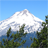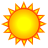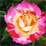# Distance between two linear features?

12687
13
01-15-2012 07:01 PMNew Contributor
I've been searching and searching for a way to calculate the minimum and mean distance from one line feature to another line feature. I'm dealing with several decades worth of shorelines for each one of my four different sites for my thesis. Currently I have a script written that cycles through each of the sites and runs an analysis from one shoreline to every other shoreline at the same site then moves on to the next year at that site and does the same thing. Right now it begins at a distance of 0.05 meters and incrementally increases a buffer around the first line until the buffer encompasses a threshold percentage of the second shoreline. I understand that this is somewhat repetitive, but that is ok. I would rather include distances for both directions. So my question is: is there a clean way to calculate the minimum and/or average distance from one line feature to another line feature? If so, how might this work? It seems like a fairly straightforward concept to calculate a set of distance statistics from one line to another (yes, I would like direction to matter as it is important to me if A to B is not the same as B to A for some reason), but I cannot seem to find how to implement this. Any help here would be much appreciated.

-Phil
------------------------
Michigan State University
Department of Geography
Tags (3)
1 Solution

Accepted SolutionsbyEsri Regular Contributor
To measure the "mean" distance between two lines could involve calculating distances from vertices of one line to the other line, and then take the average of the total. So, you can try the following:

1. For line1, use Feature Vertices To Points tool (Data Management toolbox - Features toolset; ArcInfo license) with the ALL option to get all the vertices of line1; let's call it vertices1
2. Use the Near tool to find the nearest distances from vertices1 to line2.
3. Use the Summary Statistics tool to get the "mean" and "minimum" of the NEAR_DIST from the result of step 2.

You can follow the similar steps to get the info for line2, if you want to compare the values in two directions.

If your line1 and line2 actually contain many connected lines, you can use Unsplie Line or Dissolve tool to "merge" connected lines into one line before the above processes; or you may need to use Case Field in step 3 to get the statistics for each line; have a look of tool help doc.

Hope this helps.
13 RepliesbyMVP Honored Contributor
The Near tool (requires ArcInfo) will calculate the distance from each line feature to the nearest in the other line feature class. If the coastlines are made of many different line features, either dissolve all of the coastlines from each coastline together or simply the sort the output of Near to get the minimum.

I don't know of any built-in tool to calculate mean distance. It seems to me you'd have to use some sort of linear referencing (don't ask me how, though), or Python, to chop your coastlines at some regular interval, then use Near and Summary Statistics to find the distance from each segment to the nearest point on the other coastline.Regular Contributor III
This might help give you some ideas: http://forums.arcgis.com/threads/13832-Create-Composite-Line?p=45205&viewfull=1#post45205

Another way would be to use a raster approach - with really small cells. Something like EuclideanDistannce: http://help.arcgis.com/en/arcgisdesktop/10.0/help/index.html#//009z0000001p000000.htmbyEsri Regular Contributor
To measure the "mean" distance between two lines could involve calculating distances from vertices of one line to the other line, and then take the average of the total. So, you can try the following:

1. For line1, use Feature Vertices To Points tool (Data Management toolbox - Features toolset; ArcInfo license) with the ALL option to get all the vertices of line1; let's call it vertices1
2. Use the Near tool to find the nearest distances from vertices1 to line2.
3. Use the Summary Statistics tool to get the "mean" and "minimum" of the NEAR_DIST from the result of step 2.

You can follow the similar steps to get the info for line2, if you want to compare the values in two directions.

If your line1 and line2 actually contain many connected lines, you can use Unsplie Line or Dissolve tool to "merge" connected lines into one line before the above processes; or you may need to use Case Field in step 3 to get the statistics for each line; have a look of tool help doc.

Hope this helps.New Contributor

Hi,

I have a similar problem. I want to calculate the distance of point data from 2 lines. I use the near tool for one line and it calulates the distnace and adds it to the attribute table then I calculate it for second line and it overwrites the values in the attribute table. If I simulateously calculate proximity using near to both lines it calculate only distance to nearest line not both lines. Any help would be appreciated.

Thanks

JulianNew Contributor
Thanks. That is exactly what I was looking for. This should help refine my analysis a ton. Thanks for the suggestions and help.

-Phil

To measure the "mean" distance between two lines could involve calculating distances from vertices of one line to the other line, and then take the average of the total. So, you can try the following:

1. For line1, use Feature Vertices To Points tool (Data Management toolbox - Features toolset; ArcInfo license) with the ALL option to get all the vertices of line1; let's call it vertices1
2. Use the Near tool to find the nearest distances from vertices1 to line2.
3. Use the Summary Statistics tool to get the "mean" and "minimum" of the NEAR_DIST from the result of step 2.

You can follow the similar steps to get the info for line2, if you want to compare the values in two directions.

If your line1 and line2 actually contain many connected lines, you can use Unsplie Line or Dissolve tool to "merge" connected lines into one line before the above processes; or you may need to use Case Field in step 3 to get the statistics for each line; have a look of tool help doc.

Hope this helps.byEsri Regular Contributor
you are welcome. I am glad to be able to help.  :)  Good luck to your analysis.New Contributor
Dan,

Great method described above.
Is there any way to get the 90 degree distance between points and lines? Near outputs the angle and instead of giving me the distance to nearest line segment, I would like perpendicular distance to line segment.
Any thoughts?

Thanks!
ShawnbyEsri Regular Contributor
The Near tool adds a NEAR_DIST field and optionally the NEAR_X, NEAR_Y, NAER_ANGLE fields to your input. Each NEAR_DIST value in this field is the shortest (not necessarily perpendicular) distance from a point to a line. The perpendicular line from a point to a line may intersect the line but the extension of the line. Can you first confirm that you want the perpendicular distance?

If you need the perpendicular distance from a point to a straight line, that woud be a different story. You would need to find the formular that represents the straight line using the coordinates of the line, and then calculate the perpendicular distance from the point (xy) to the line. Have a look of here:
http://www.intmath.com/plane-analytic-geometry/perpendicular-distance-point-line.phpNew Contributor
I am interesting in the perpendicular distance between a pt and a line and it seems that based on the link you sent (which makes intuitive sense!), the shortest distance between a pt and a line is necessarily perpendicular.
But you suggested that the shortest distance between a pt and a line is not necessarily perpendicular. Can you explain?